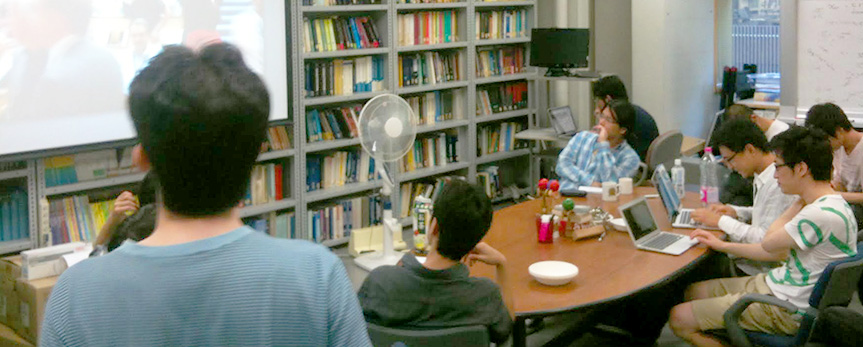Theoretical Particle Physics (A1)

Research Areas:
Quantum Field Theory, Particle Phenomenology, Cosmology, Superstring Theory, Mathematical Physics, Quantum Foundation
Overview:
In A1 subcourse (Theoretical Particle Physics), we conduct theoretical research to address a fundamental question, what is the origin of the space-time and matter. Some concrete examples of our focus are; Model building beyond the Standard model; Particle cosmology, such as dark matter and inflation; Superstring, M-theory, quantum gravity, supersymmetric gauge theory; Mathematical physics related to QFT such as duality and integrability; Quantum foundation -- the conceptual and mathematical underpinnings of the quantum theory.